GSAK (Geocaching Swiss Army Knife)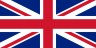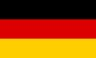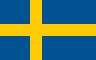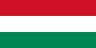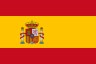Contents - Index

DateDiff (function)

DateDiff(dDate1,dDate2) : Number

Calculates the number of days between two dates. If result is 0 then they are the same Date, if result is negative then Date2 occurs before Date1. Today and yesterday are 1 day apart.

Example: Calculate the number of days between 1st January, 2013 and 31st August 2013:

 \$diff = DateDiff(,) msgok msg=\$diff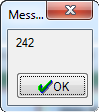Related: StringToDate() DateFormat() DateToString() DateToSql()

Alpha List         Category List